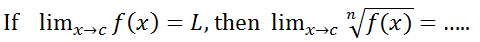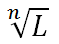Question FBQ1 : A ___ is a phenomenon that relates one variable or quantity to other variables or quantities

Question FBQ2 : The___ of the function is the set of all values taken on by the dependent variable

Question FBQ3 : In the equation, $y=4{x}^{2}+3$, $x$ is the ___ variable

Question FBQ4 : To decide whether an equation defines a function, it is helpful to isolate the ___on the left

Question FBQ5 : The equation $y=±\sqrt{1-{x}^{2}}$ indicates that $y=+1$ or ­­­­­­­­___________

Question FBQ6 : The solution of when $x=1$ is ___

Question FBQ8 : The value of $f\left(x\right)=2{x}^{3}-4x+1$ when $x=0$ is ___

Question FBQ9 : If p is a polynomial function and c is any real number, then $\underset{x\to c}{\mathrm{lim}}p\left(x\right)=___$

Question FBQ10 : In sketching the graph of a function, we allow the horizontal axis represent the ___ variable

Question FBQ11 : A function is ___ if every horizontal line intersects the graph of the function at most once

Question FBQ12 : If two values of $y$ corresponds to an $x$ value, then it is said that ___
Answer: y is not a function of x

Question FBQ13 : The function given by $\left(f.g\right)\left(x\right)=f\left(g\left(x\right)\right)$ is the ___ of $f$ with $g$

Question FBQ14 : If $f\left(x\right)=2x+1$ and $g\left(x\right)={x}^{2}+2$ the value of $g\left(f\left(x\right)\right)$ is ___
Answer: 4x2 + 4x + 3

Question FBQ15 : Given that $f\left(x\right)=\sqrt{x}$ and , $f\left(g\left(1\right)\right)$ = ___

Question FBQ16 : In an inverse function, the domain of $f$ must be equal to the range of ${f}^{-1}$, and the range of $f$ must be equal to the domain of ___

Question FBQ17 : The inverse function of $f\left(x\right)={x}^{3}$ is ___

Question FBQ18 : $f$ has no inverse if does not pass the ________ test

Question FBQ19 : For a continuous function $\underset{x\to {x}_{0}}{\mathrm{lim}}f\left({x}_{0}\right)$ =

Question FBQ20 : $\underset{x\to -2}{\mathrm{lim}}\frac{5}{x+2}=___$

Question FBQ21 : Let c be a real number and $f\left(x\right)=g\left(x\right)$ for all $x\ne c$. If the limit of $g\left(x\right)$ exists as $x\to c$, then the limit of $f\left(x\right)$ also exists and $\underset{x\to 2}{\mathrm{lim}}f\left(x\right)=\underset{x\to 2}{\mathrm{lim}}g\left(x\right)$. This theorem is known as the ___

Question FBQ22 : Evaluating $\underset{x\to 1}{\mathrm{lim}}\frac{{x}^{3}-1}{x-1}$ gives ___

Question FBQ23 : A polynomial function is continuous at every real ___

Question FBQ24 : There are ___ categories of discontinuities

Question FBQ25 : A function is said to be continuous if and only if it is continuous at every point of its ___

Question FBQ26 : The function $f\left(x\right)=\sqrt{3-x}$ is continuous in the interval ___

Question FBQ27 : Continuity of a function is expressed some times by saying if the x value are closed together, then the ___values of the function will also be close

Question FBQ28 : Derivative is often described as the “___ rate of change”

Question FBQ29 : The process of finding a derivative is called ___

Question FBQ30 : ___ is defined as the ratio of the vertical distance the line rises or falls between two points P and Q to the horizontal distance between P and Q

Question FBQ31 : ___ theorem can be used to find the derivative of $y={x}^{n}$

Question FBQ32 : The derivative of $\mathrm{cot}x$ is ___

Question FBQ33 : The derivative of is ___

Question FBQ34 : If we differentiate ${a}^{x}$, we get ___

Question FBQ35 : If $y=uv$, then the product rule is given as $\frac{dy}{dx}$ ___

Question FBQ36 : The quotient rule for differentiation is given as $\frac{dy}{dx}$ ___

Question FBQ37 : The chain rule of differentiation is given as___ $\frac{dy}{dx}$

Question FBQ38 : The derivative of $y=tan\left(3x+2\right)$ is ___

Question FBQ39 : The derivative of $y=sin\left(4x+3\right)$ is ___

Question FBQ40 : $xy+cosy=5$ is an example of an___ function

Question FBQ41 : If , $\frac{dy}{dx}$ gives ___

Question FBQ42 : The $c$ in integration is called an ___ constant

Question FBQ43 : The general formula for integration $\int {x}^{n}dx$ is given as ___

Question FBQ44 : $\int \frac{-1}{\sqrt{1-{x}^{2}}}dx$

Question FBQ45 : $\int \frac{1}{\sqrt{{x}^{2}-1}}dx=$

Question FBQ46 : $\int \frac{1}{\sqrt{{x}^{2}+1}}dx=$

Question FBQ47 : $\int \frac{{f}^{\text{'}}\left(x\right)}{f\left(x\right)}dx=$

Question FBQ48 : The volume of a cone ${V}_{cone}=$___

Question FBQ49 : The volume of a sphere is given as ${V}_{x}=$ ___

Question FBQ50 : $\int {a}^{x}dx=$

Question FBQ7 : The equation of the tangent at x = 2 on the curve is ___
Answer: 5y + 2x – 14 = 0

Question MCQ1 : Which of the equation below define y as a function of x?
Answer: ${x}^{2}+y=1$

Question MCQ2 : Find $f\left(-1\right)$ for the equation $y={2x}^{2}-4x+1$

Question MCQ3 : Find the value of $f\left(x\right)=1-5x+{x}^{2}$ for $x=4$

Question MCQ4 : Let $f\left(x\right)={x}^{2}-4x+7$, find $\frac{f\left(x+∆x\right)-f\left(x\right)}{∆x}$
Answer: $2x+∆x-4$

Question MCQ5 : Division by Zero is...

Question MCQ6 : A function is said to be one to one if...
Answer: To each value of the dependent variable in the range, there corresponds exactly one value of the independent variable

Question MCQ7 : If $f\left(x\right)=2x-4$ and $g\left(x\right)={x}^{2}+3$, find $f\left(g\left(x\right)\right)$
Answer: $2\left({x}^{2}-1\right)$

Question MCQ8 : If ${f}^{-1}\left(x\right)=x-8$, then $f\left(x\right)=$?
Answer: $x+8$

Question MCQ9 : Let f $\left(x\right)=\frac{1}{x}$ , then ${f}^{-1}\left(x\right)$ is given as...
Answer: $x$

Question MCQ20 : Which of the following conditions does NOT determine the continuity of $f$ at point c?
Answer: $\underset{x\to c}{\mathrm{lim}}f\left(c\right)=f\left(x\right)$

Question MCQ21 : $f\left(x\right)=\frac{\left({x}^{2}-1\right)}{\left(x-1\right)}$ is continuous on the interval ...

Question MCQ22 : Discontinuities fall into two categories namely ...

Question MCQ23 : The representation $\underset{x\to {b}^{-}}{\mathrm{lim}}f\left(x\right)=f\left(b\right)$ shows that ...
Answer: $f$ is continuous from the left at b

Question MCQ24 : Which of the following statements is true about the continuity of $f\left(x\right)=\sqrt{3-x}$
Answer: $f$ is continuous on the interval $\left(-\infty ,3\right]$

Question MCQ25 :  Which of the following about the continuity of is NOT correct?
Answer: $\underset{x\to 2}{\mathrm{lim}}g\left(x\right)$ is continuous on the interval $\left[-\infty ,\infty \right]$

Question MCQ26 : Differentiate $y={x}^{3}+5{x}^{2}-4x+2$ with respect to $x$
Answer: $3{x}^{2}+10x-4$

Question MCQ27 : Find the value of $\frac{dy}{dx}$ at $x=2$, if $y={x}^{4}+6{x}^{3}-4{x}^{2}+7x-2$

Question MCQ28 : The derivative of $sinx+cosx=\dots$
Answer: $cosx-sinx$

Question MCQ29 : What is the derivative of ?
Answer: $1/x$

Question MCQ30 : If the derivative of $\mathrm{sec}x=\mathrm{sec}x\mathrm{tan}x$, find the derivative of

Question MCQ31 : If we differentiate ${e}^{x}$, we get ...
Answer: ${e}^{x}$

Question MCQ32 : Find the derivative of $y={x}^{3}sinx$
Answer: $x\left({x}^{2}cosx+3sinx\right)$

Question MCQ33 : Differentiate $y={x}^{2}{\left(2x-5\right)}^{4}$ with respect to $x$ yields ...
Answer: $2x{\left(2x-5\right)}^{3}\left(6x-5\right)$

Question MCQ34 : Differentiate with respect to $x$ if $y=\frac{sinx}{{x}^{2}}$
Answer: $\frac{xcosx-2sinx}{{x}^{3}}$

Question MCQ35 : The gradient of a curve is found ...
Answer: At a point on the curve

Question MCQ36 : In differentiation, the chain rule technique is used when differentiating ...
Answer: A function of a function

Question MCQ37 : Find $\int \left({s}^{3}+4s\right)ds$
Answer: $\frac{{s}^{4}}{4}+2{s}^{2}+c$

Question MCQ38 : Evaluate $\int {\left(3x-2\right)}^{6}dx$
Answer: $\frac{{\left(3x-2\right)}^{7}}{21}+c$

Question MCQ39 : Evaluate $\int cos\left(6x+4\right)dx$
Answer: $\frac{sin\left(6x+4\right)}{6}+c$

Question MCQ40 : Evaluate $\int {\left(sec}^{2}8x\right)dx$

$\frac{tan8x}{8}+c$

Question MCQ41 : Find $\int \frac{2x-5}{{x}^{2}-5x+6}dx$
Answer: $\mathrm{ln}\left({x}^{2}-5x+6\right)+c$

Question MCQ42 : Find $\int cotxdx$

$\mathrm{ln}sinx+c$

Question MCQ43 :  Evaluate $\int x{e}^{2x}dx$ using integration by parts

Question MCQ44 : Definite integrals can also be used to calculate ...

Question MCQ45 : The infinitesimal volume of a cylinder representing an element of integration revolving around the $x$ axis is given by ...
Answer: ${V}_{x}=\pi -\underset{a}{\overset{b}{\int }}{y}^{2}dx$

Question MCQ46 : The infinitesimal volume of a cylinder representing an element of integration revolving around the $y$ axis is given by ...
Answer: ${V}_{y}=\pi \underset{a}{\overset{b}{\int }}{x}^{2}dx$

Question MCQ47 : Find the volume of a sphere generated by a semicircle $y=\sqrt{{r}^{2}-{x}^{2}}$ revolving around the $x-axis.$

Question MCQ48 : Find the volume of a right circular cone generated by the line (segment) passing through the origin and point $\left(h,r\right)$, where $h$ denotes the height of the cone and $r$ is the radius of its base revolving around the $x-axis$.
Answer: ${V}_{cone}=\frac{1}{3}\pi {r}^{2}h$

Question MCQ49 : Find the value of $dy/dx$ for $y={\left(2x+5\right)}^{3}$ at $x=4$

Question MCQ50 : What is the value of $\int cosxdx$ at $x=30$?
Answer: $\frac{1}{2}$

Question MCQ15 : If , then $\underset{x\to c}{\mathrm{lim}}\left[bf\left(x\right)\right]=bL$ is called...

Question MCQ16 : Find $\underset{x\to 2}{\mathrm{lim}}{\left(x}^{2}+2x-3\right)$
Answer: $5$

Question MCQ17 : Evaluate $\underset{x\to 1}{\mathrm{lim}}f\left(x\right)$ if
Answer: $3$

Question MCQ18 : Find $\underset{x\to 0}{\mathrm{lim}}f\left(x\right)$ if
Answer: $1}{2}$

Question MCQ19 : Let , then = ...

Question MCQ10 : Find the inverse function of $f\left(x\right)=\sqrt{2x-3}$
Answer: $\frac{{x}^{2}+3}{2}$

Question MCQ11 : Find the domain and range of $f\left(x\right)=4-{x}^{2}$, leaving your result in interval notation.
Question MCQ13 : Find the limit: $\underset{x\to 1}{\mathrm{lim}}f\left(x\right)$ if
Question MCQ14 : Evaluate $\underset{x\to 1}{\mathrm{lim}}\left({x}^{2}+1\right)$
Question MCQ12 :Answer: